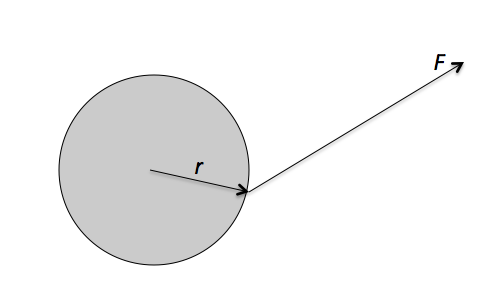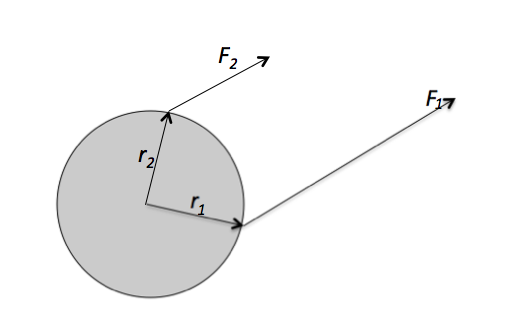Classical Mechanics

# Torque as a Cross Product

A force $\vec{F} = \left( 7 \hat{i} + 8 \hat{k} \right) \text{ N}$ is applied to a rotating object. If the displacement vector from the pivot to the point of action is $\vec{r} = 3 \hat{i} \text{ m},$ what is the torque?

Consider two vectors $\vec{r} = 3 \hat{j} \text{ m}$ and $\vec{F} = \left( 6 \hat{i} + 9 \hat{k} \right) \text{ N}.$ What is the torque in unit vector form?A force of $\vec{F} = \left(3 \hat{i} + 6 \hat{j} \right) \text{ N}$ is applied to a disc, as shown in the figure above. The displacement vector from the center of the disc to the force's point of action is $\vec{r} = \left( 7 \hat{i} + 3\hat{j} \right) \text{ m}.$ Find the torque produced by the force $\vec{F}.$Forces $\vec{F_1} = \left( 6 \hat{i} + 9 \hat{j} \right) \text{ N}$ and $\vec{F_2} = \left( 9 \hat{i} + 14 \hat{j} \right) \text{ N}$ are applied to a disc, as shown in the figure above. The displacement vectors from the center of the disc to the points of action of forces $\vec{F_1}$ and $\vec{F_2}$ are $\vec{r_1} = 9 \hat{i} \text{ m}$ and $r_2 = 5 \hat{j} \text{ m},$ respectively. Find the net torque produced by the forces.

A plum is located at $( 9 \text{ m}, 12 \text{ m} )$ on the $xy$-coordinate plane. If a force of $\vec{F} = ( 4 \text{ N} , 8 \text{ N} )$ is applied to the plum, what is the torque relative to the origin $O?$

×